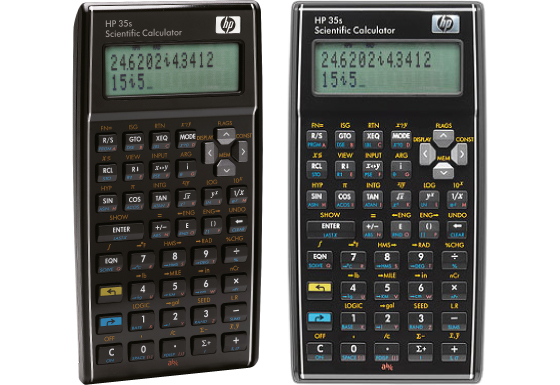## HP 35s

The HP 35s offers a unique way to switch between RPN and algebraic entry-system logic and is completely programmable, or you can use some of over 100 built-in functions. The clear 2-line display with adjustable contrast allows not only easy entry but also browsing history of previous calculations.Specification

• Built-In Functions: 100+
• Entry System: Algebraic (D.A.L.), RPN
• Power Source: Battery (CR2032 × 2)
• Dimensions and Weight: 152 × 82 × 18 mm, 125 g

Mathematical Functions

• Fractions
• Equation Solver (Newton)
• System of Linear Equations
• Matrix and Vector Calculations
• Numeric Derivative and Integral
• Complex Number Calculation

Statistics

• 1-Variable Statistics
• 2-Variable Statistics
• Regression Calculations
• Mean, Standard Deviation
• nCr, nPr, n!
• Random Numbers

Number Systems

• Number Systems: DEC, BIN, OCT, HEX, PEN
• Logical Operation (AND, OR, NOT, NEG, XOR)

Memory

• Multi-Line Playback
• Memory Variables: 800

Notation and Decimal Places

• FSE Display (SCI, FIX, ENG)

Trigonometric Functions